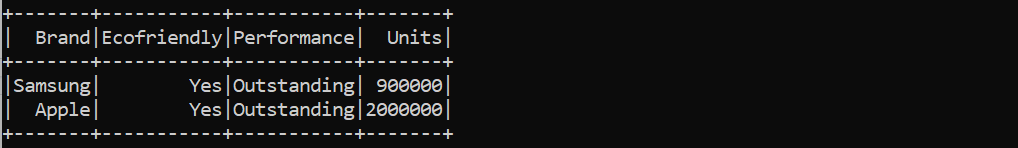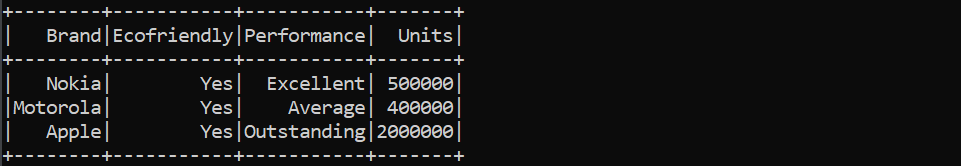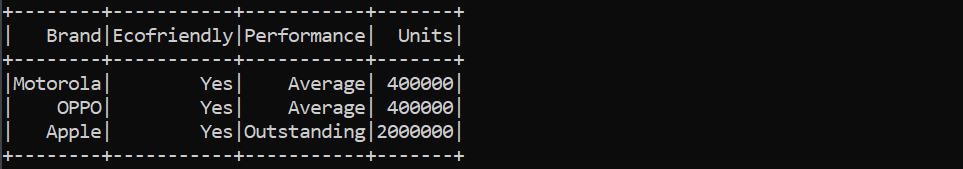# Simple random sampling and stratified sampling in PySpark

• Last Updated : 16 May, 2022

In this article, we will discuss simple random sampling and stratified sampling in PySpark.

## Simple random sampling:

In simple random sampling, every element is not obtained in a particular order. In other words, they are obtained randomly. That is why the elements are equally likely to be selected.  In simple words, random sampling is defined as the process to select a subset randomly from a large dataset. Simple random sampling in PySpark can be obtained through the sample() function. Simple sampling is of two types: replacement and without replacement. These types of random sampling are discussed below in detail,

## Method 1: Random sampling with replacement

Random sampling with replacement is a type of random sampling in which the previous randomly chosen element is returned to the population and now a random element is picked up randomly.

Syntax:

sample(True, fraction, seed)

Here,

• fraction: It represents the fraction of rows to be generated.  It might range from 0.0 to 1.0 (inclusive)
• seed: It represents the seed required sampling (By default it is a random seed). It is used to regenerate the same random sampling.

Example:

## Python3

 `# Python program to demonstrate random``# sampling in pyspark with replacement` `# Import libraries``import` `pandas as pd``from` `pyspark.sql ``import` `Row``from` `pyspark.sql ``import` `SparkSession`  `# Create a session``spark ``=` `SparkSession.builder.getOrCreate()` `# Create dataframe by passing list``df ``=` `spark.createDataFrame([``    ``Row(Brand``=``"Redmi"``, Units``=``1000000``, Performance``=``"Outstanding"``, Ecofriendly``=``"Yes"``),``    ``Row(Brand``=``"Samsung"``, Units``=``900000``, Performance``=``"Outstanding"``,  Ecofriendly``=``"Yes"``),``    ``Row(Brand``=``"Nokia"``, Units``=``500000``, Performance``=``"Excellent"``,  Ecofriendly``=``"Yes"``),``    ``Row(Brand``=``"Motorola"``,Units``=``400000``, Performance``=``"Average"``,  Ecofriendly``=``"Yes"``),``    ``Row(Brand``=``"Apple"``, Units``=``2000000``,Performance``=``"Outstanding"``,  Ecofriendly``=``"Yes"``)``])` `# Apply sample() function with replacement``df_mobile_brands ``=` `df.sample(``True``, ``0.5``, ``42``)` `# Print to the console``df_mobile_brands.show()`

Output:## Method 2: Random sampling without replacement

Random sampling without replacement is a type of random sampling in which each group has only one chance to be picked up in the sample.

Syntax:

sample(False, fraction, seed)

Here,

fraction: It represents the fraction of rows to be generated.  It might range from 0.0 to 1.0 (inclusive)

seed: It represents the seed required sampling (By default it is a random seed). It is used to regenerate the same random sampling.

Example:

## Python3

 `# Python program to demonstrate random``# sampling in pyspark without replacement` `# Import libraries``import` `pandas as pd``from` `pyspark.sql ``import` `Row``from` `pyspark.sql ``import` `SparkSession` `# create the session``spark ``=` `SparkSession.builder.getOrCreate()` `# Create dataframe by passing list``df ``=` `spark.createDataFrame([``    ``Row(Brand``=``"Redmi"``, Units``=``1000000``, Performance``=``"Outstanding"``, Ecofriendly``=``"Yes"``),``    ``Row(Brand``=``"Samsung"``, Units``=``900000``, Performance``=``"Outstanding"``,  Ecofriendly``=``"Yes"``),``    ``Row(Brand``=``"Nokia"``, Units``=``500000``, Performance``=``"Excellent"``,  Ecofriendly``=``"Yes"``),``    ``Row(Brand``=``"Motorola"``,Units``=``400000``, Performance``=``"Average"``,  Ecofriendly``=``"Yes"``),``    ``Row(Brand``=``"Apple"``, Units``=``2000000``,Performance``=``"Outstanding"``,  Ecofriendly``=``"Yes"``)``])` `# Apply sample() function without replacement``df_mobile_brands ``=` `df.sample(``False``, ``0.5``, ``42``)` `# Print to the console``df_mobile_brands.show()`

Output:## Method 3: Stratified sampling in pyspark

In the case of Stratified sampling each of the members is grouped into the groups having the same structure (homogeneous groups) known as strata and we choose the representative of each such subgroup (called strata). Stratified sampling in pyspark can be computed using sampleBy() function. The syntax is given below,

Syntax:

sampleBy(column, fractions, seed=None)

Here,

• column: the column that defines strata
• fractions: It represents the sampling fraction for every stratum. When the stratum is not given, we assume fraction as zero.
• seed: It represents the seed required sampling (By default it is a random seed). It is used to regenerate the same random sampling.

Example:

In this example, we have three strata, 1000000, 400000, and 2000000 and they are selected according to the fractions, 0.2, 0.4, and 0.2 respectively.

## Python3

 `# Python program to demonstrate stratified sampling in pyspark` `# Import libraries``import` `pandas as pd``from` `pyspark.sql ``import` `Row``from` `pyspark.sql ``import` `SparkSession` `# Create the session``spark ``=` `SparkSession.builder.getOrCreate()` `# Creating dataframe by passing list``df ``=` `spark.createDataFrame([``    ``Row(Brand``=``"Redmi"``, Units``=``1000000``, Performance``=``"Outstanding"``, Ecofriendly``=``"Yes"``),``    ``Row(Brand``=``"Samsung"``, Units``=``1000000``, Performance``=``"Outstanding"``,  Ecofriendly``=``"Yes"``),``    ``Row(Brand``=``"Nokia"``, Units``=``400000``, Performance``=``"Excellent"``,  Ecofriendly``=``"Yes"``),``    ``Row(Brand``=``"Motorola"``,Units``=``400000``, Performance``=``"Average"``,  Ecofriendly``=``"Yes"``),``    ``Row(Brand``=``"OPPO"``,Units``=``400000``, Performance``=``"Average"``,  Ecofriendly``=``"Yes"``),``    ``Row(Brand``=``"Apple"``, Units``=``2000000``,Performance``=``"Outstanding"``,  Ecofriendly``=``"Yes"``)``])` `# Applying sampleBy() function``mobile_brands ``=` `df.sampleBy(``"Units"``, fractions``=``{``  ``1000000``: ``0.2``, ``2000000``: ``0.4``, ``400000``: ``0.2``}, seed``=``0``)` `# Print to the console``mobile_brands.show()`

Output:My Personal Notes arrow_drop_up